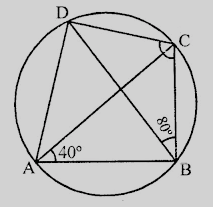# $ABCD$ is a cyclic quadrilateral in which $\angle DBC = 80^o$ and $\angle BAC = 40^o$. Find $\angle BCD$.

Given:

$ABCD$ is a cyclic quadrilateral in which $\angle DBC = 80^o$ and $\angle BAC = 40^o$.

To do:

We have to find $\angle BCD$.

Solution:

$ABCD$ is a cyclic quadrilateral.

Join diagonals $AC$ and $BD$.$\angle DBC = 80^o, \angle BAC = 40^o$

Arc $DC$ subtends $\angle DBC$ and $\angle DAC$ in the same segment

Therefore,

$\angle DBC = \angle DAC = 80^o$

$\angle DAB = \angle DAC + \angle CAB$

$= 80^o + 40^o$

$= 120^o$

$\angle DAC + \angle BCD = 180^o$               (Sum of opposite angles of a cyclic quadrilateral is $180^o$)

$120^o +\angle BCD = 180^o$

$\angle BCD = 180^o- 120^o$

$= 60^o$

Hence $\angle BCD = 60^o$.

Updated on: 10-Oct-2022

28 Views